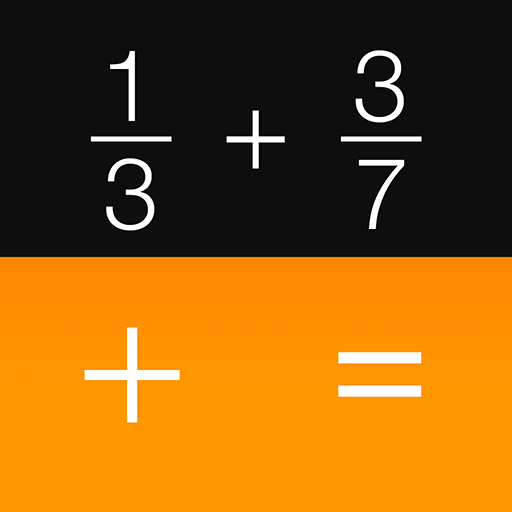# Fraction Calculator + Decimals

##### About Fraction Calculator + Decimals
Introducing the world’s first Fraction Calculator packed with additional functions like reducing or simplifying fractions, fractions-to-decimals and a decimals-to-fractions calculator. All these in one nifty app. Discover an easy way to solve everyday fraction problems. Add, subtract, multiply, divide and even convert fractions, quickly and clearly. Visual Math Interactive’s Fraction Calculator is an excellent homework help and business reference tool, with nice clean keypads and large display type for your fast and easy calculations.

FEATURES:
- Fraction calculator app and decimal-to-fractions app in one.
- Also automatically reverse-converts fractions to decimals for your quick reference.
- Supports improper and proper fractions, mixed numbers and whole numbers.
- Now you can also go in reverse manner: Calculate from Decimals to Fractions as well.
- Big, clear, uncluttered keypads for quick and easy calculations, every time.
- Bonus Homework Help: Need extra help understanding fractions? Now, you can also stream FREE visually interactive Fraction Basics videos to learn the basics of fractions quickly.

YOU MIGHT ALSO NEED:
Zap Zap Fractions
The fun way to learn the basics of fractions, enhanced with interactive visualization, gamification, and performance analytics.

Fraction Basics
A comprehensive refresher course on fractions in 12 easy, visual, search-friendly animated videos. Also dubbed: “The dictionary of fractions.”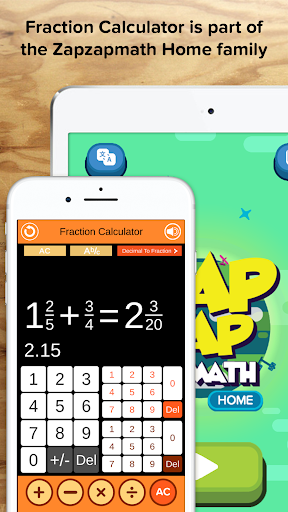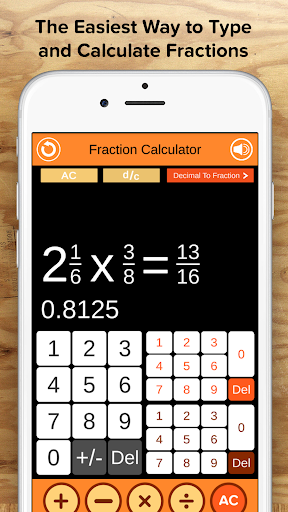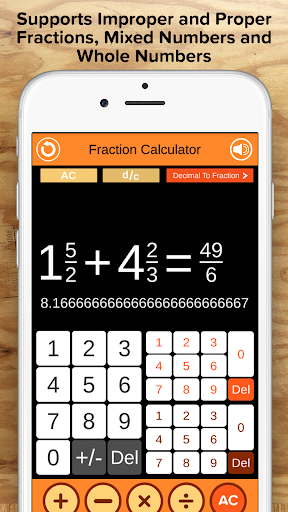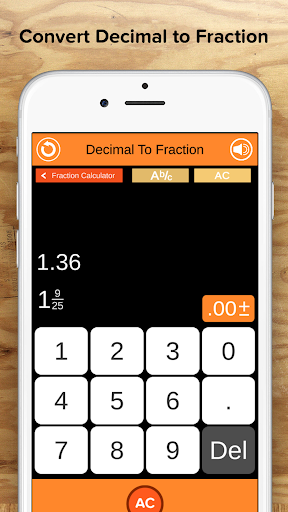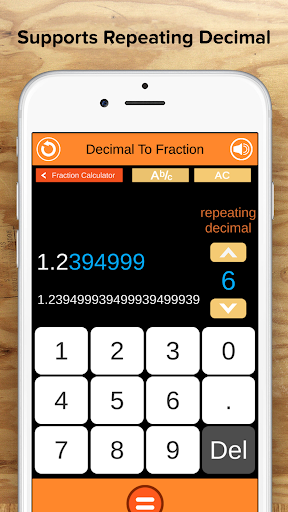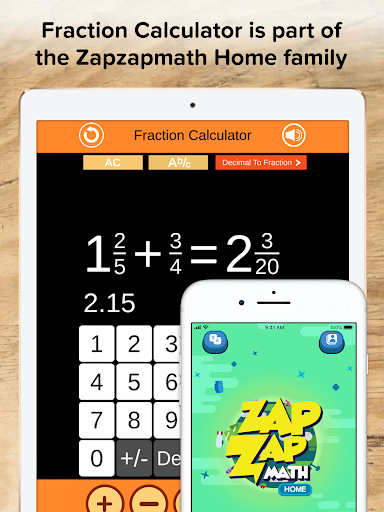##### Recent Updates - Version 2.1.4
• - Fixed some bugs